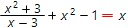# Solve equations onlineGenerally, the equation of some variable x is of the form:

f(x) = 0

To solve the equation above, one should find all the values of variable x such as f(x) = 0 is the correct identity.

Graphically, the roots of some equation f(x) = 0 are the abscisses of the intersection points of function f(x) with axis x:Therefore, from the above plot of some function f(x), we immediately can conclude that the values x1, x2, x3, x4 are the roots of equation f(x) = 0.

There are infinity number of different equations (linear, quadratic, cubic, trigonometric, equations with powers and radical, ect.) depending on concrete form of function f(x).

Our online calculator is based on Wolfram Alpha LLC system. It is able to find roots of many different forms of equations with step by step solution.

Solve equationsEquation you want to solve: